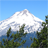cancel
Showing results for
Did you mean:

1,036 ViewsContributor V

hello, I am using S12ZVML128 controller.

I am not getting what i have missed in this code to get the conversion value.

volatile unsigned short ADC0ResultList = {0};
volatile unsigned short ADC1ResultList = {0};

typedef enum
{

{

ADC0CTL_0 = 0x09; // single access mode-data bus,Trigger mode
//ADC0CTL_0_STR_SEQA = 1; // Store result at abort/restart
ADC0FMT_DJM = 1; // left justified result data
ADC0FMT_SRES = 4; // 12-bit result
ADC0TIM=0; // clock: clk = fbus / (2x(reg.value + 1)) [0.25 - 8MHz]
ADC1CTL_0 = 0x09; // single access mode-data bus,Trigger mode
//ADC1CTL_0_STR_SEQA = 1; // Store result at abort/restart
ADC1FMT_DJM = 1; // left justified result data
ADC1FMT_SRES = 4; // 12-bit result
ADC1TIM=0; // clock: clk = fbus / (2x(reg.value + 1)) [0.25 - 8MHz]

}

{
UINT8 lu8cmdListVar=0;
UINT8 var=0;
{
{

}
}
{
{

}
}
else
{

}

}

Labels (1)
• ### General

5 Replies
386 ViewsNXP Employee

Hi PRATIBHA,

I shortly checked your code and currently I cannot see any obvious issue except the potential issue with command and result list alignment.

volatile UINT8 ADC0CommandList __attribute__ ((aligned (4))) = {{0xC0,0x00,0x00,0x00}};

volatile UINT8 ADC1CommandList __attribute__ ((aligned (4))) = {{0xC0,0x00,0x00,0x00}};

volatile unsigned short ADC0ResultList __attribute__ ((aligned (4))) = {0};

volatile unsigned short ADC1ResultList __attribute__ ((aligned (4))) = {0};

These lists must be aligned to 4byte boundary due to internal DMA transfer mechanism.

I hope it helps you.

Have a great day,

-----------------------------------------------------------------------------------------------------------------------
-----------------------------------------------------------------------------------------------------------------------

386 ViewsContributor V

Hi,I want to calculate the conversion time for that GetADCValue(E_ADC_CHANNEL_NUMBER leAdcChanNo) function.Could you please tell me,how much time it will take to execute? I am using clock settings as follows,

crystal frequency=20Mhz

#define _EXTERNAL_CLOCK

void InitClock(void)
{
while (GDUF_GLVLSF)
GDUF_GLVLSF = 1;

CPMUREFDIV_REFDIV = 3;
CPMUREFDIV_REFFRQ = 1;
CPMUSYNR_SYNDIV = 4;
CPMUSYNR_VCOFRQ = 1;
CPMUPOSTDIV_POSTDIV = 1;
#if 1
// Oscillator clock monitor reset is enabled
CPMUOSC2_OMRE = 1;
#endif
#ifdef _EXTERNAL_CLOCK
CPMUOSC_OSCE = 1;
while (CPMUIFLG_UPOSC == 0) {}; // Wait for oscillator to start up (UPOSC=1) and PLL to lock (LOCK=1)
#endif

while (CPMUIFLG_LOCK == 0) {};
CPMURFLG = 0x60; //Clear PORF and LVRF
}

386 ViewsNXP Employee

Hi PRATIBHA,

When #ifdef _EXTERNAL_CLOCK

fREF = fOSC/(REFDIV + 1) = 20MHz/(3+1) = 5MHz

fVCO = 2 * fREF * (SYNDIV + 1) = 2*5MHz*(4+1)=50MHz

fPLL = fVCO/(POSTDIV + 1) = 50MHz/(1+1) = 25MHz

fBUS = fPLL/2 = 25MHz/2 = 12.5MHz

else

fREF = fIRC1M = 1MHz  //incorrect REFFRQ value

fVCO = 2 * fREF * (SYNDIV + 1) = 2*1MHz*(4+1)=10MHz //invalid fVCO, it has to be at least 32MHz

fPLL = fVCO/(POSTDIV + 1) = 10MHz/(1+1) = 5MHz

fBUS = fPLL/2 = 5MHz/2 = 2.5MHz

So, the #ifdef _EXTERNAL_CLOCK condition may be dangerous in your code. I would like to recommend add #else with PLL parameters for internal IRC clock as source.

Now, I let’s assume that you have fBUS = 12.5MHz.

Maximum fATDCLK is 8.33MHz

So, fATDCLK= fbus / (2x(reg.value + 1)) = 6.25MHz

The ADC Conversion Period for 12bit is 19~39 ADC clocks, where we will calculate with 19 due to shortest sample time.

ADC Conversion Period = 19/fATDCLK = 3.04us.

I hope it helps you.

Have a great day,

-----------------------------------------------------------------------------------------------------------------------
-----------------------------------------------------------------------------------------------------------------------

386 ViewsContributor V

I have calculated the GetADCValue function execution time, its taking 9us to execute and inner while loop is taking 5us.

I want to reduce this conversion time.Please suggest me for that.

386 ViewsContributor V

Than you very much,now my code is working.